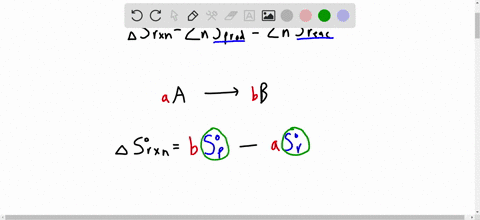Enroll in one of our FREE online STEM summer camps. Space is limited so join now!View Summer Courses### How can $\Delta S^{\circ}$ be relatively independ…

04:41Need more help? Fill out this quick form to get professional live tutoring.

Get live tutoring
Problem 49

With its components in their standard states, a certain reaction is spontaneous only at high $T$ . What do you know about the signs of $\Delta H^{\circ}$ and $\Delta S^{\circ} ?$ Describe a process for which this is true.

Check back soon!

## Discussion

You must be signed in to discuss.

## Video Transcript

So this question we have to think about what Adults? Jesus. So Delta G is, by definition, Delta aged minus T Delta s. And if Delta G is less than zero, it's spontaneous. And if Delta G is greater than zero, the reactions and on spontaneous. And so in this scenario, we're told that at high tea, so is temperature goes up, the reaction becomes spontaneous is to say g become zero. My apartment is becomes less than zero. So if as temperature goes up, this becomes more negative, this is the term that's temperature. This means that the tea Delta s term has to be over. All this has to be positive since it's being subtracted. So as it gets larger and makes Delta G go toward more negative. And so if this is all very positive, temperature has been positive. Delta s has to be great. Zero. And so that tells us the information about Delta's adults. Asked now if we're told that it goes from being non spontaneous to being spontaneous, which it says that it is, that means that a low temperature, basically, when this term it doesn't really count. Let's just say T equals 11 Kelvin like this is this turn doesn't play a rule. That means Delta G is non spontaneous. It has to be ground zero, so it's only depending on its age. So Delta H has to also hear it. So that's what we know for this reaction.